Highway Materials

# Highway Materials - Notes | Study Transportation Engineering - Civil Engineering (CE)

 1 Crore+ students have signed up on EduRev. Have you?

Group Index of Soils (G.I)

In order to classify the fine-grained soils within one group and for judging their suitability as subgrade material, an indexing system has been introduced in HRB classification which is termed as group index. Soils are thus assigned arbitrary numerical numbers known as group index (GI). Group index is function of percentage and is given by the equation G.I = 0.2a + 0.005ac + 0.01bd
Where,Where, WL = Liquid limit, IP = Plasticity Index.
P = Percentage fines passing from 0.074 mm sieve.
0 ≤ G . I ≤ 20 Lower the group → best quality.

Plate Bearing Test

(i)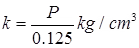Here,
k = Modulus of subgrade reaction
P = Pressure Corresponding to settlement of 0.125 cm.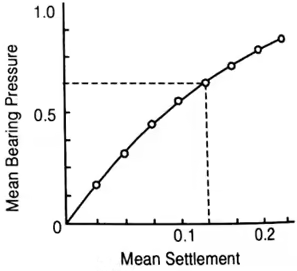(ii)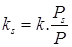Where,
ks = Modulus of subgrade reaction for soaked condition.
Ps = Pressure required in the soaked condition to produce same deformation as deformation Produce by pressure ‘P’ in consolidated condition.
k = Modulus of subgrade reaction for the consolidated stage.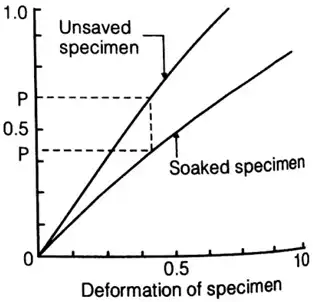Δ = Deformation in ‘cm’.
a = Radius of rigid plate in ‘cm’.
k = P / Δ = E / 1.18a
E = Modulus of elasticity of soil subgrade in kg/cm2.
k.a = constant
a1 = Radius of smaller plate (other plate)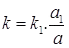k1 = Modulus of subgrade reaction of other plate of radius ‘a1’ cm.

California Bearing Ratio (CBR) Test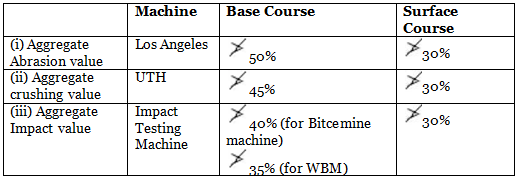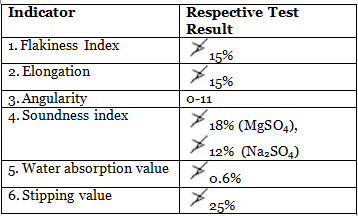(i) Aggregate crushing test
Aggregate crushing value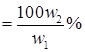Where, w1 = Weight of the test sample in ‘gm’
w2 = weight of the crushed material in ‘gm’ passed through 2.36 mm sieve.

(ii) Shape Tests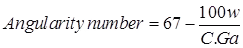Where, Ga = specific gravity of aggregate
W = mass of mould containing aggregate
C = mass of mould containing water
(iii) Abrasion Test
(a) Los Angeles Abrasion Test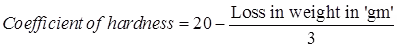Bituminous Material

1. Product of fractional distillation of Petroleum: Gasoline, Naptha, Kerosene, Lubricating oil and Residue – Petroleum Bitumen.
2. Cutback Bitumen: Reduced Viscosity Bitumen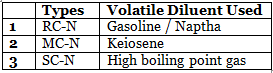N – Numeral [0, 1, 2, 3, 4, 5]
Show progressive thickening from 0 to 5
3. Specific Gravity:
Bituminous → 0.97 – 1.02
Tar → 1.1 – 1.5
1. Bituminous Mixes
(i) Determination of Specific GravityWhere, Ga = Average specific gravity of blended aggregate mix.
w1, w2, w3, w4 are % by weight of aggregate 1, 2, 3 & 4 respectively. G1, G2, G3 & G4 are specific gravities of the aggregate 1, 2, 3 & 4 respectively.
(ii) Specific Gravity of Compacted Specimen
(a)Where, Gt = Theoretical maximum specific gravity of the mix.
Wb = % by weight of bitumen.
Gb = Specific gravity of bitumen.
Ga = Average specific gravity of aggregates.
(b) Theoretical density γt, per-cent solids by volume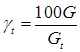Where, G = Actual specific gravity of test specimen
Gt = Theoretical maximum specific gravity.
(c) Voids in the Mineral Aggregate (VMA)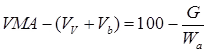Where, Vb = % of bitumen
Wa = Aggregate content percent by weight
Vv = % air voids in the specimen.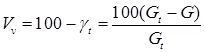(d) % Voids Filled with Bitumen (VFB)
2. Marshall Method Bituminous Mix Design
Percentage Air Voids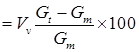Where, Gm = Bulk density or mass density of the specimen
Gt = Theoretical specific gravity of mixtureWhere,
W1 = Percent by weight of coarse aggregate in total mix
W2 = Percent by weight of fine aggregate in total mix
W3 = Percent by weight of filler in total mix
W4 = Percent by weight of bitumen in total mix
G1 = Apparent specific gravity of coarse aggregate
G2 = Apparent specific gravity of fine aggregate
G3 = Apparent specific gravity of filter
G4 = Specific gravity of bitumen
Percent Voids in Mineral Aggregate (VMA)
VMA = Vv + Vb
Here, Vv = Volume of air voids, %
Vb = Volume of bitumen,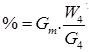Per-cent Voids Filled with Bitumen (VFB)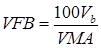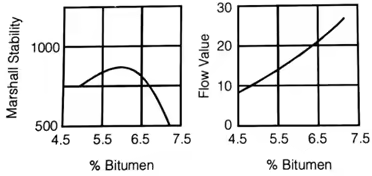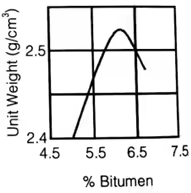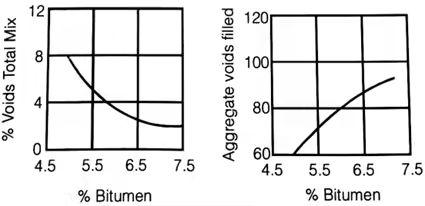HveeM Method of Bituminous mix Design Stabilimeter Value, (s)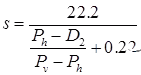Where, Pv = Vertical pressure at 28 kg/cm2 or at a total load of 2268 kg.
Ph = Horizontal pressure corresponding to Pv = 28 kg/cm2.
D2 = Displacement on specimen represented as number of turns of pump handle to raise Ph from 0.35 to 7 kg/cm2.

Cohesiometer Value, (c)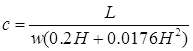Where, L = Weight of shots in cm.
w = Diameter of width of specimen in cm
H = Height of specimen in cm

Stabilometer Resistance R-valuewhere, Pv = Vertical pressure applied (11.2 kg/cm2)
Ph = Horizontal pressure transmitted at Pv = 11.2 kg/cm2.
D2 = Displacement of stabilometer fluid necessary to increase the horizontal pressure from 0.35 to 7kg/cm2, measured in number of revolutions of the calibrated pump handle.

The document Highway Materials - Notes | Study Transportation Engineering - Civil Engineering (CE) is a part of the Civil Engineering (CE) Course Transportation Engineering.
All you need of Civil Engineering (CE) at this link: Civil Engineering (CE)

## Transportation Engineering

22 videos|68 docs|39 tests
 Use Code STAYHOME200 and get INR 200 additional OFF

## Transportation Engineering

22 videos|68 docs|39 tests

Track your progress, build streaks, highlight & save important lessons and more!

,

,

,

,

,

,

,

,

,

,

,

,

,

,

,

,

,

,

,

,

,

;